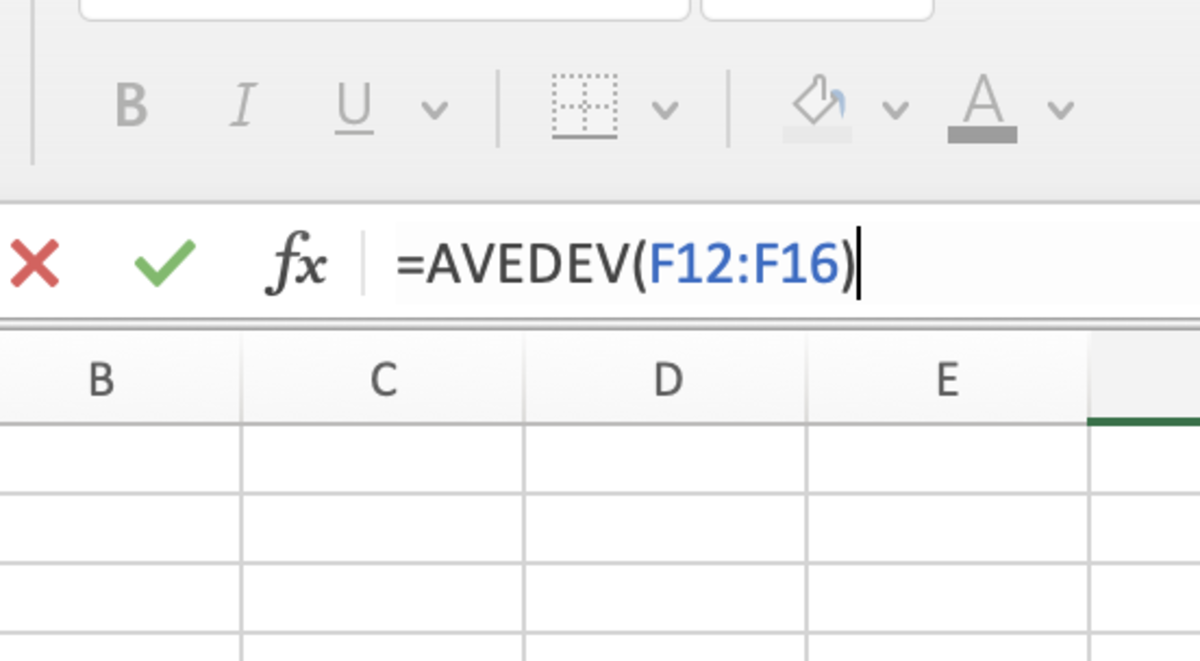# How to Use the AVEDEV Function in Excel for Mac

Joshua earned an MBA from USF and he writes mostly about software and technology.The AVEDEV function gives an Excel user the ability to analyze variability of a data set.Pexels

## The Purpose of the AVEDEV Function

The AVEDEV function gives an Excel user the ability to analyze variability of a data set by returning the average of absolute deviations between data points and their collective mean.

The mean absolute deviation or mean deviation can be accurately defined in the formula below with a sample of data.

While the mean deviation is important to descriptive statistics, it's not frequently used in statistics education or practice due to its complexities.

## The AVEDEV Function Syntax

The AVEDEV function needs to be inputted into a cell like a formula for it work. To manually add this function, the following steps need to be taken:

1. A cell needs clicked type "=AVEDEV(".
2. After the open parenthesis the array of the data set needs to be added followed by a closed parenthesis.
3. After the formula is created the formula can be entered.

Below in bold the syntax of the AVEDEV function.

AVEDEV(number1, [number2], ...)

This formula can compute up to 255 numbers. Individual numbers or cells can be used when separated by a comma, but it's generally more efficient to use an array of data with this formula.The AVEDEV function gives an Excel user the ability to analyze variability of a data set.

## AVEDEV Examples

There are several examples of the AVEDEV function in use in the illustration below. There are four methods used that all produce the same result.

## Inserting the AVEDEV Function

The AVEDEV function can be inserted into a cell by first selecting the cell, then clicking in the formulas tab. Next, click on the other functions button and the statistical option. Select AVEDEV from from the list of functions.

Scroll to Continue

Once the formula builder appears, numbers can be added to see the results at the bottom of this sidebar. This formula builder can be a great help to reference characters.

In addition, all methods shown in the example above can be used in the formula builder. After the arguments are added, the done button can be clicked for the function to be entered into the cell.

Microsoft. (n.d.). AVEDEV function. Retrieved July 21, 2022, from https://support.microsoft.com/en-us/office/avedev-function-58fe8d65-2a84-4dc7-8052-f3f87b5c6639#:~:text=Description,variability%20in%20a%20data%20set.

Wolfram Mathworld. (n.d.). Mean Deviation. Retrieved July 21, 2022, from https://mathworld.wolfram.com/MeanDeviation.html

How to Use the COUNT Function in Excel

How to Use the AVERAGE Function in Excel

How to Use the ABS Function in Excel

How to Remove Errors in Excel With the IFERROR Function

How to Use the VLOOKUP Function in Excel

Converting Measurement Units: Using the CONVERT Function

To learn more about using functions in Excel I recommend The Excel 2019 Bible book. I have been using the Excel Bible for years to improve my understanding of all aspects of this Microsoft product.

This content is accurate and true to the best of the author’s knowledge and is not meant to substitute for formal and individualized advice from a qualified professional.